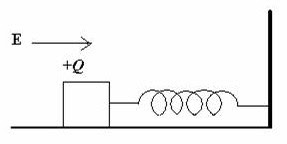## Thursday, April 28, 2011

### IIT-JEE 2011 Questions (MCQ) on Simple Harmonic Motion

“Do not worry about your problems with mathematics, I assure you mine are far greater.”

– Albert Einstein

The following two questions on simple harmonic motion were included in the IIT-JEE 2011 question paper. Both questions are simple for those who have mastered the fundamental principles:(1) A wooden block performs SHM on a frictionless surface with frequency, ν0. The block carries a charge +Q on its surface. If now a uniform electric field E is switched on as shown, then the SHM of the block will be

(A) of the same frequency and with shifted mean position

(B) of the same frequency and with the same mean position

(C) of changed frequency and with shifted mean position

(D) of changed frequency and with the same mean position

When the uniform electric field is switched on, the positively charged block experiences a constant force in the direction of the electric field and so the mean position of the block is shifted. But the frequency (ν) of oscillation of the block is unchanged since it depends only on the mass m of the block and the force constant k of the spring, in accordance with the relation

ν = (1/2π) [√(k/m)]

The correct option is (A).

(2) A point mass is subjected to two simultaneous sinusoidal displacements in x-direction, x1(t) = A sin ωt and x2(t) = A sin(ωt + 2π/3). Adding a third sinusoidal displacement x3(t) = B sin(ωt + φ) brings the mass to a complete rest. The value of B and φ are

(A) √2 A, 3π/4

(B) A, 4π/3

(C) √3 A, 5π/6

(D) A, π/3

The particle in the above question is subjected to two simultaneous simple harmonic motions (SHM) of the same frequency and amplitude in the same direction. the resultant motion of the particle is simple harmonic as is evident by adding x1(t) and x2(t):

x1(t) + x2(t) = A sin ωt + A sin(ωt + 2π/3) = A sin(ωt +π/3)

The amplitude of the resultant simple harmonic motion is A itself but the initial phase of the motion is now π/3.

As the particle remains at rest on adding the third simple harmonic motion, x3(t) = B sin(ωt + φ) the amplitude (B) of the third SHM must be A itself, but it must be 180º (or, π radian) out of phase. In other words, the initial phase (φ) of the third simple harmonic motion must be π/3 + π = 4π/3

The correct option is (B).

You can find a useful post on simple harmonic motion here.